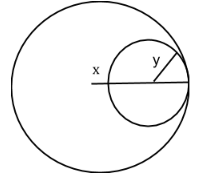Courses
Courses for Kids
Free study material
Free LIVE classes
MoreLIVE
Join Vedantu’s FREE Mastercalss

# Two circles touch internally. The sum of their areas is $116\pi cm{}^\text{2}$and the distance between their centres is 6 cm. Find the radius of circles.A) 8cm, 20cmB) 4cm, 10cmC) 6cm, 8cmD) 5cm, 9cmVerified
361.2k+ views
Hint: To solve the question, we have to represent the given data in a diagram which hints the relation between the radius of the circles. Apply relevant formulae to solve the equation and find out the values.

Let the radius of two circles be $x,y$ where $x>y$.The distance between the centre of the circles given = 6 cm.
From the diagram we observe that the distance between the centre of the circles = x - y
$\Rightarrow x-y=6$ ……. (1)
We know that the formula of area of the circle $=\pi {{r}^{2}}$
Where r is the radius of the circle.
Thus, the sum of the areas of the circles with radius x, y $=\pi {{x}^{2}}+\pi {{y}^{2}}$.
The sum of the areas of the circles given $=116\pi cm{}^\text{2}$.
$\Rightarrow \pi {{x}^{2}}+\pi {{y}^{2}}=116\pi cm{}^\text{2}$
$\pi \left( {{x}^{2}}+{{y}^{2}} \right)=116\pi$
${{x}^{2}}+{{y}^{2}}=116$
By substituting the equation (1) in the above equation, we get
${{\left( 6+y \right)}^{2}}+{{y}^{2}}=116$ ……. (2)
We know that the formula for ${{\left( a+b \right)}^{2}}$ is given by ${{a}^{2}}+{{b}^{2}}+2ab$
On comparing the expression ${{\left( a+b \right)}^{2}}$with ${{\left( 6+y \right)}^{2}}$, we get a = 6 and b = y
By substituting the above formula in equation (2), we get that
${{6}^{2}}+2(6)y+{{y}^{2}}+{{y}^{2}}=116$
$36+12y+{{y}^{2}}+{{y}^{2}}=116$
$2{{y}^{2}}+12y-116+36=0$
$2{{y}^{2}}+12y-80=0$
$2\left( {{y}^{2}}+6y-40 \right)=0$
${{y}^{2}}+6y-40=0$
${{y}^{2}}+10y-4y-40=0$
$\left( y+10 \right)\left( y-4 \right)=0$
Thus, the possible values of y = 4 cm, -10 cm.
Radius is a measurement which cannot be negative.
$\Rightarrow$y = 4cm
The value of x = 6 + y = 6 + 4 = 10cm.
Thus, the radius of the circles are 4 cm,10 cm.
Hence the option (b) is the correct answer.

Note: The possibility of mistake is the calculations while solving the quadratic equations. The alternative method to solve the question is by option elimination method, from the given information we understand that the difference between the radius of the circles is equal to 6 cm. Thus, we can arrive at the solution while eliminating the other three options.
Last updated date: 28th Sep 2023
Total views: 361.2k
Views today: 10.61k